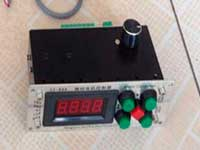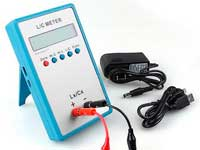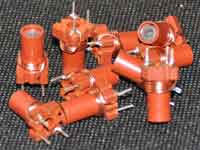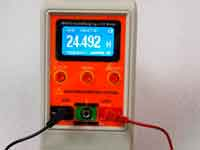Q-factor - 4.3 out of 5 based on 4 votes
Rating 4.25 (4 Votes)

About Q-factor of RF inductance coilCalculation of Q-factor inductor is quite complex. Ultimately, the quality factor depends on many factors - the loss in the wires, in core,  in shield. Accurate accounting of theese all are very difficult. However, we can simplify the problem, if we take into account only the losses in the wire. Firstly, they are major contributors to the total amount of losses, and secondly estimate the quality factor of the coil we are interested under creating more high Q circuits. In this case, we are applying the special actions to minimize the losses by the way of implementing a ribbing core or an air-core inductance coil.

I remind you that the quality factor of inductance coil is the ratio of the reactance coil (2πƒL) to its loss resistance. Determine the reactance of the coil is not difficult. But the assessment of loss in the wire is not so simple and we will view it in detail ...Search the solution led to the site G3YNH - Dr. David W Knight. He carried out extended theoretical research on the calculation of the impedance at high frequencies, the calculation of inductors, the choice of formulas approximating the experimental measurements. They can be found in the site chapter - "From Transmitter to Antenna". Article devoted to the calculation of the coils here. These studies formed the basis of the method of calculation of Q-factor in Coil32. Special thanks to D.Knight.

One of the first who tried to take into account the losses in the inductance coils at radio frequencies was S.Butterworth, in the work: "Effective Resistance of inductance Coils at Radio Frequencies, 1926". He is more famous as the designer of well-known "Butterworth filters". But the Butterworth method calculates the losses in wire on RF with high accuracy only for infinitely long solenoid.

The losses in the conductor at high frequencies consist of three factors:

1. First of all, depending on the material of the wire. It is obvious that a wire of a metal with a high resistivity will have larger losses. As is well known the wire-resistance to DC can be calculated as:
 RDC = ρl/A. где A = πr2 
• RDC – DC Resistance [Ohm]
• ρ – resistivity metal [Ohm · m] (for example silver = 1.59 · 10-8 ohm-m)
• l – length of wire [m]
• A – conductor cross-section [m2]
• r – radius of the wire [m]
It can be argued that this is the basic losses in the wire and the loss at high frequencies can not be less than the losses at the DC.
2.The skin effect phenomenon is where alternating current that flowing on the surface of the wire shields the inner layers and avoid itself to travel to the center of a conductor, in result in almost all the current is concentrated in a thin surface layer whose thickness is determined by the following expression:
 δi = √[ ρ / ( π f μ )] 
• δi – skin depth [m], the depth at which the current density is reduced by a factor e (e = 2.71 - Euler number)
• ρ – resistivity metal [Ohm · m]
• f – frequency [Hz]
• μ = μ0r, μ0 = 4π · 10-7 - absolute permeability, μr - relative permeability of the metal (close to unity for most nonmagnetic metals)
It is obvious that the impedance for alternating current increases with frequency, as skin depth decreases. For convenience, logical calculations, we introduce the concept of the coefficient of skin effect - Ξ. It will be a factor indicating how many times the resistance of conductor for the alternating current at a given frequency higher DC resistance.
 RAC = RDC · Ξ 
Classic method calculates the area of the ring from surface to skin depth Aeff = π (2rδi - δi2) and inserts it in the formula , calculating the AC resistance instead DC. The skin factor in this case is expressed as:
 Ξ = r²/( 2rδi - δi²) 
Physically, this formula can be expressed as the ratio of the DC conductivity (proportional to r2) to the AC conductivity (proportional to r and δi). David Knight improve this method for more accurate AC resistance formulae. See reference below...
3.Availability phenomenon proximity effect. The effect is that the magnetic field from the adjacent turns of the wire coil induces eddy currents. The superposition of these currents with currents from the skin effect causes that the current density of the conductor fragment that located in inner part of the solenoid is higher and skin is not similar to the ring and resembles a crescent.

Obviously, the AC impedance under the influence of the proximity effect is further increased. The effect decreases with the decreasing the proximity, ie by increasing the gap between the turns. It should be borne in mind that as the proximity effect and skin effect are two aspects of the same phenomenon - the interaction of RF current with the magnetic field.

Due to the extreme complexity of description of the electromagnetic field associated with the RF coils there are no simple theoretically based approximate formulas for the calculation of the proximity effect in arbitrarily constructed radio frequency coil. We can use the computer model in electromagnetic simulators that use finite element method - COMSOL Multiphysics, FEMM, ANSYS, etc. But to simplify the calculations we can use psychoanalytical calculation methods using tables compiled on the basis of experimental measurements. We go down that way.

By analogy with the skin factor we introduce the concept of proximity factor - Ψ, then:

 RAC = RDC · Ξ · Ψ 
The proximity effect is not exists in a straight conductor, and in this case Ψ = 1.

In 1947, radio engineer R.G.Medhurst - member of Research Laboratory of "General Electric Co.Ltd." published a number of works related to experimental studies inductors, including studies of the proximity effect. The results of these studies are relevant to this day. From the measurements of parameters of real coils Medhurst came to conclusion that the Butterworth method does not work for short coils. The term "short" refers to coil with the ratio l / D <7 and winding pitch is less than twice diameter of the wire. (ie exactly those that most often used in practice).
We are interested in the experimental data on Ψ. Medhurst leads them in a table in dependence on the relation of coil length to diameter ratio (l / D) and the winding pitch to the wire diameter (p / d). Yellow marked coincidence between experiment and theory Butterworth on error less than 3%:

 p/d → l/D ↓ 1 1.111 1.25 1.429 1.667 2 2.5 3.333 5 10 0 5.31 3.73 2.74 2.12 1.74 1.44 1.2 1.16 1.07 1.02 0.2 5.45 3.84 2.83 2.2 1.77 1.48 1.29 1.19 1.08 1.02 0.4 5.65 3.99 2.97 2.28 1.83 1.54 1.33 1.21 1.08 1.03 0.6 5.8 4.11 3.1 2.38 1.89 1.6 1.38 1.22 1.1 1.03 0.8 5.8 4.17 3.2 2.44 1.92 1.64 1.42 1.23 1.1 1.03 1 5.55 4.1 3.17 2.47 1.94 1.67 1.45 1.24 1.1 1.03 2 4.1 3.36 2.74 2.32 1.98 1.74 1.5 1.28 1.13 1.04 4 3.54 3.05 2.6 2.27 2.01 1.78 1.54 1.32 1.15 1.04 6 3.31 2.92 2.6 2.29 2.03 1.8 1.56 1.34 1.16 1.04 8 3.2 2.9 2.62 2.34 2.08 1.81 1.57 1.34 1.165 1.04 10 3.23 2.93 2.65 2.27 2.1 1.83 1.58 1.35 1.17 1.04 ∞ 3.41 3.11 2.815 2.51 2.22 1.93 1.65 1.395 1.19 1.05

This table can be represented as an array of data and the intermediate data be obtained by the method of linear interpolation. This was done in the online calculator ON4AA - http://hamwaves.com/antennas/inductance.html. Perhaps this is the single calculator that considers the quality factor correctly. The Coil32_v11.7.0.900 uses the method from the work: "The resistance of round-wire single-layer inductance coils by A.H.M.Arnold, D.Eng., Associate Member 1951". The same method is used in Android version of Coil32. The method is based on the formulas Butterworth and lists with correction factors for short coils and calculates proximity effect factor with an accuracy of ± 10%. This takes into account the working frequency, the number of turns of the coil, material of the wire and that is not possible to do directly by Medhurst table. The program has the opportunity to choose the material of the wire. In real design of air core inductance coil the deviation of calculations from the measurement can reach 20-30%.There is an optimum ratio of the winding pitch to the diameter of the wire depending on the form factor of the coil. Medhurst gives this dependence in the form of a graph.

If we consider that the highest quality factor for the coil is reached with l / D ≈ 1, in this case the optimal ratio of the coil winding pitch to diameter ratio p / d ≈ 2, ie we can uniquely determine the optimum diameter of the wire, knowing the geometry of the coil. Moreover, the coil with the same form factor (l / D ≈ 1), has the minimum parasitic capacitance. This is understandable because the coil with such winding geometry has a minimum length of wire with a maximum inductance. Such optimal coils are geometrically similar, ie the all dimentions of the coil including wire diameter we may to increase and its self-resonant frequency will be decreased, about the same as adopted in antenna technology. But the Q-factor of theese coils not will be differ.

References:

Сomments from anonymous guests are enabled with moderation.

Coil Winding MachineUS \$187.53
The system consists of individual components including a controller, a stepping motor, a foot switch, power adapter and output shaft. This design makes it very easy for you to integrate the system into your own project.

LC Meter LC200AUS \$37.99
Measuring Capacitance Range:
0.01 pF - 100mF
Measuring Inductance Range:
0.001 uH - 100H
Measurement accuracy:1%.

RF InductorsUS \$6.43
Variable Ferrite Core Inductor coil set 3.5Turns 25-100MHZ

Auto Ranging LCR MeterUS \$63.07
Meter can measure 0.00pF to 100.00mF capacitance, 0.000uH to 100.00H inductance and 0.00? to 10.000M? resistance. Very suitable for small value capacitor and inductor measurement.